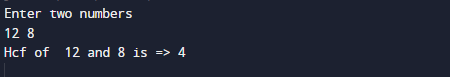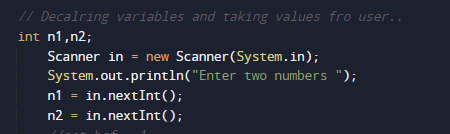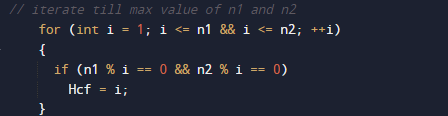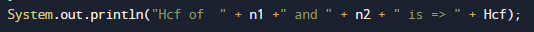# Java Program to find GCD of two numbers

In this tutorial you will learn about the Java Program to find GCD of two numbers and its application with practical example.

In this tutorial, we will learn to create a Java Program to find GCD of two numbers using Java programming.

## Prerequisites

Before starting with this tutorial we assume that you are best aware of the following Java programming topics:

• Java Operators.
• Basic Input and Output function in Java.
• Class and Object in Java.
• Basic Java programming.
• If-else statements in Java.
• For loop in Java.

## What Is GCD?

GCD(Greatest Common Divisor) or HCF (Highest Common Factor) . When  we finding the factor of two numbers, Highest Common Factor of  numbers is the Largest factor that divides these two numbers. The greatest factor found from the common factors is called the HCF. It is also called the Greatest common factor.

### Methods of Greatest Common Factor.

• Note all the factors of two number.
• Fetch the common factors.

Example: Find HCF 12 and 8.
Factors of 12=> 1, 2, 3, 4, 6, 12.
Factors of 8=> 1, 2, 4, 8
Common Factors: 1, 2, 4
Greatest Common Factor: 4
Then HCF of 12 and 8 is = >4.

## Java Program to find GCD of two numbers

In this program we will find GCD of two given number using  loops. We would first declared and initialized the required variables. Next, we would prompt user to input two numbers.Later we will find the GCD of given number.

## OutputIn the above program, we have first declared and initialized a set variables required in the program.

• n1 =It will hold first number given by user.
• n2 =It will hold second number given by user.
• Hcf= it will hold Greatest common divisor.

After that we take a numbers from user and find greatest common divisor between them.Here, we take two numbers from user whose HCF are to be found are stored in n1 and n2 respectively.

Here loop will be executed until i is less than both “n1 & n2” in that way all number between 1 and smallest of two are iterated to find HCF.If both first  and second number are divisible by i,Hcf is set to the particular number. This will continue until we finds the largest number (HCF) which divides both First and second number without any  remainder as shown in image above.

And Finally we will Print Hcf of both the number.In this tutorial we have learn about the Java Program to find GCD of two numbers and its application with practical example. I hope you will like this tutorial.